## A tool for Calculating the geodetic coordinates (Latitude, Longitude and Height) from the Cartesian Earth-Centered-Earth-Fixed (ECEF) X, Y, Z coordinates, and Calculating the ECEF Coordinates (X, Y, Z) to the geodetic coordinates (L, L, H)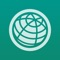# ECEF Coordinate Converter - between LLH and XYZ

by Jian Li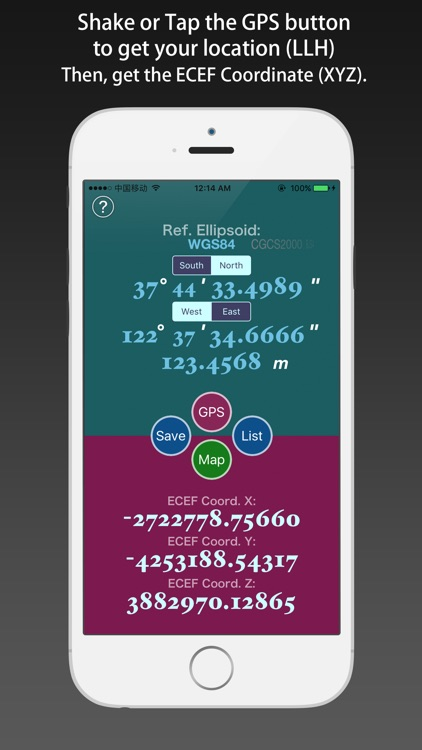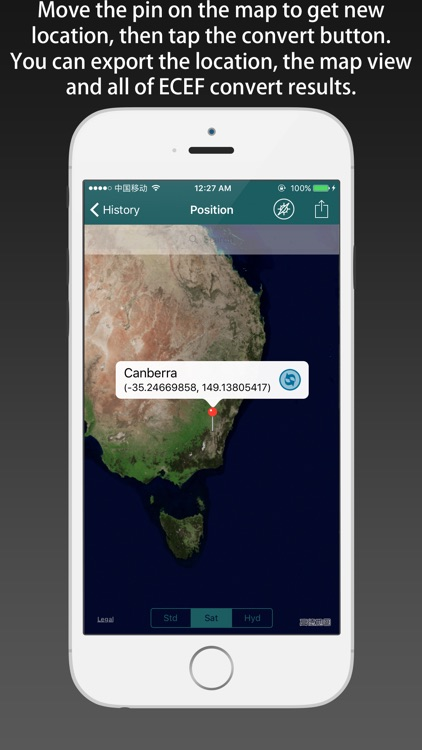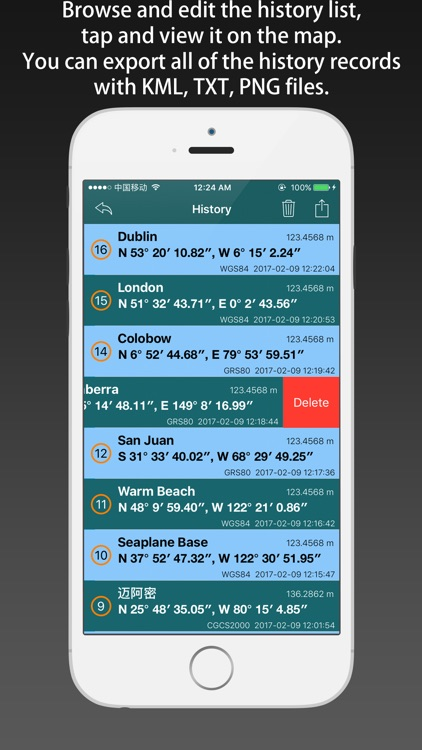A tool for Calculating the geodetic coordinates (Latitude, Longitude and Height) from the Cartesian Earth-Centered-Earth-Fixed (ECEF) X, Y, Z coordinates, and Calculating the ECEF Coordinates (X, Y, Z) to the geodetic coordinates (L, L, H).### App Details

Version
1.1
Rating
NA
Size
5Mb
Genre
Last updated
March 29, 2017
Release date
February 10, 2017

### App Screenshots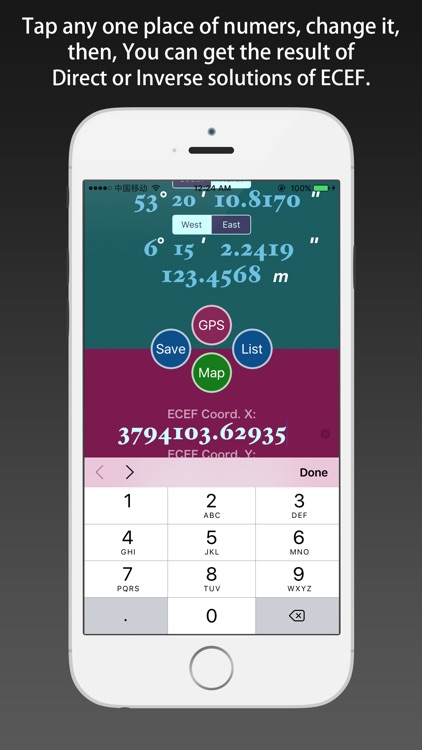### App Store Description

A tool for Calculating the geodetic coordinates (Latitude, Longitude and Height) from the Cartesian Earth-Centered-Earth-Fixed (ECEF) X, Y, Z coordinates, and Calculating the ECEF Coordinates (X, Y, Z) to the geodetic coordinates (L, L, H).

PS: NAD83 = GRS80. (Reference Ellipsoid)

Every point that is expressed in ellipsoidal coordinates can be expressed as an rectilinear X Y Z (Cartesian) coordinate. Cartesian coordinates simplify many mathematical calculations. The Cartesian systems of different datums are not equivalent.

ECEF ("earth-centered, earth-fixed"), also known as ECR ("earth-centered rotational"), is a geographic coordinate system and Cartesian coordinate system, and is sometimes known as a "conventional terrestrial" system. It represents positions as an X, Y, and Z coordinate. The point (0,0,0) is defined as the center of mass of the earth, hence the name "earth-centered." Its axes are aligned with the international reference pole (IRP) and international reference meridian (IRM) that are fixed with respect to the surface of the earth, hence the description "earth-fixed." This term can cause confusion since the earth does not rotate about the z-axis (unlike an inertial system such as ECI), and is therefore alternatively called ECR.

[Features]
1) support direct and inverse solutions (LLH to XYZ, XYZ to LLH).
2) support several types of reference ellipsoid (WGS84, GRS80(NAD83), PZ90, CGCS2000, BJ54, GDZ80 etc).
3) support using GPS to get the location, and transform it to ECEF coordinate.
4) support moving the pin on the map to obtain the latitude and longitude of the location, and transform them.
5) support saving the convertion results, and the history records can be viewed, deleted, cleaned up, and shared.
6) Every the historical record can be shown on the map, and each of them can be reduced again.

[Location Input Method]
1) using the keyboard input by hand.
2) using the GPS positioning.
3) moving the pin on the map.

[Instructions]
Direct solution (LLH -> XYZ):
1) Select the Reference Ellipsoid.
2) Tap the “GPS” button to get the location.
3) Or Input the location by keyboard.
4) Inputed the number, tap the “Done” button, then you can get the ECEF Coordinate.

Inverse solution (XYZ -> LLH):
1) Select the Reference Ellipsoid.
2) Input the ECEF Coordinate components (X, Y, Z).
3) Inputed the number, tap the “Done” button, then you can get the Geodetic Coordinate.

Disclaimer:
AppAdvice does not own this application and only provides images and links contained in the iTunes Search API, to help our users find the best apps to download. If you are the developer of this app and would like your information removed, please send a request to [email protected] and your information will be removed.